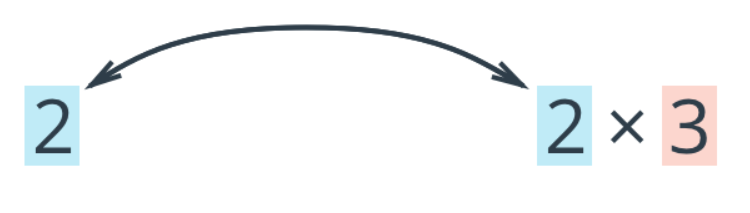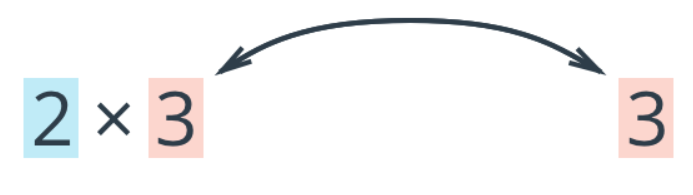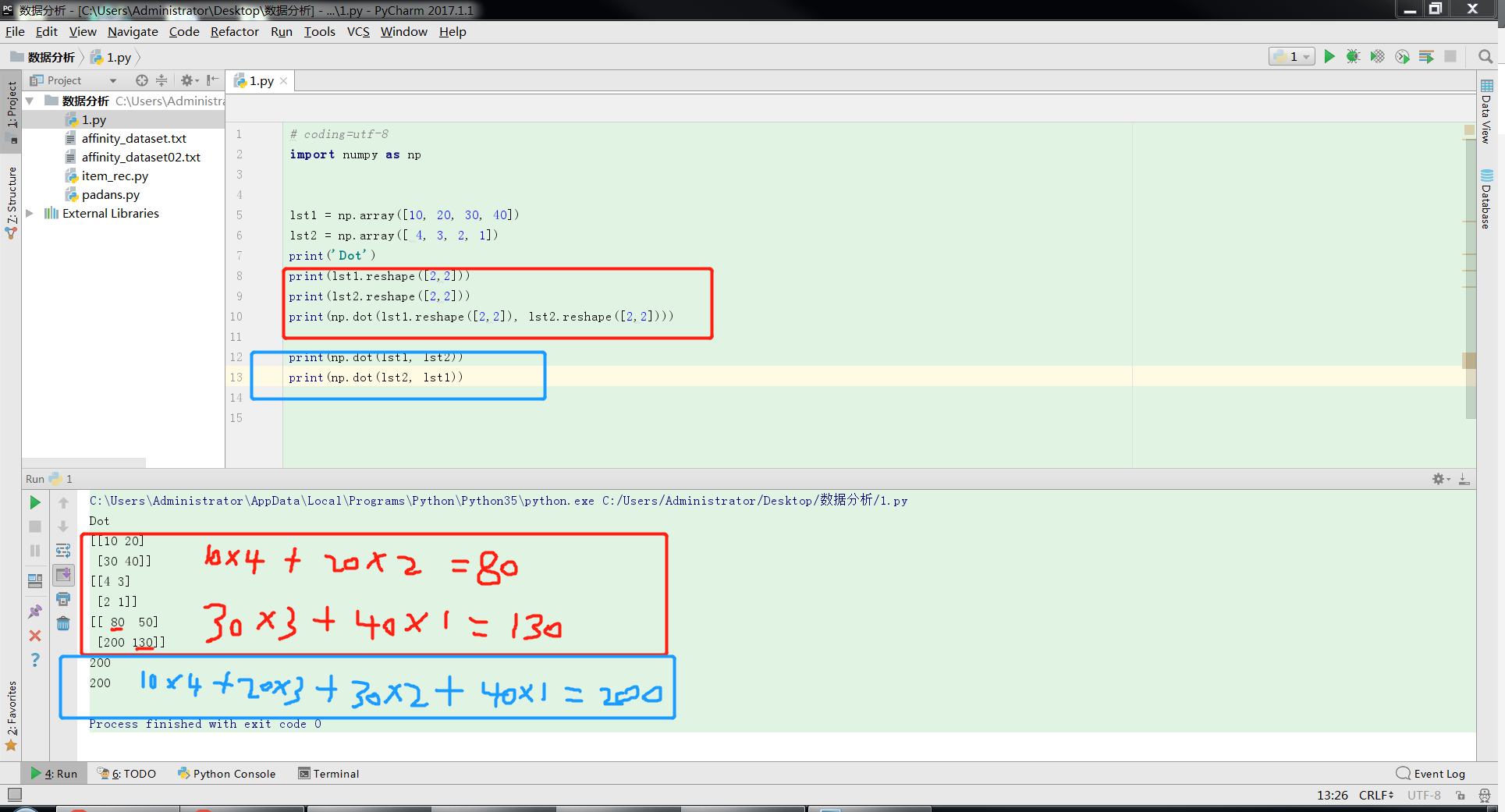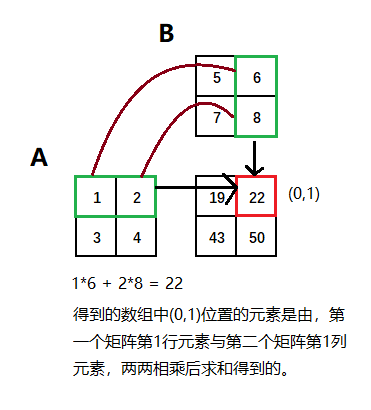• numpy 矩阵点积时，经常遇到这样的错误： ValueError: shapes (3,2) and (3,) not aligned: 2 (dim 1) != 3 (dim 0) 这表示点积左边的矩阵维度(dim) 是 3 * 2 的，而右边的数组有 3 个元素，2 != 3，于是报错。...
numpy 矩阵点积时，经常遇到这样的错误：
ValueError: shapes (3,2) and (3,) not aligned: 2 (dim 1) != 3 (dim 0)

这表示点积左边的矩阵维度(dim) 是 3 * 2 的，而右边的数组有 3 个元素，2 != 3，于是报错。这时可以将右边的数组移到点积的左边，于是变成了 3 个元素的数组和 3 * 2 的矩阵的点积，此时 3 = 3，便不会报错了。数组和矩阵做点积时，需要相邻的数字相等，否则就会报上面的错误。numpy.ndarray 进行星乘时，如果维度对不上，提示的错误则如下所示：
ValueError: operands could not be broadcast together with shapes (3,) (2,2)

关于 点积 和 星乘 之间的区别，参考文章numpy 中 的 星乘(*) 和 点乘(.dot) 点积 和 向量乘法（外积）
展开全文• NumPy中dot()函数的理解 今天学习到numpy基本的运算方法，遇到了一个让我比较难理解的问题。就是dot函数是如何对矩阵进行运算的。 一、dot()的使用 ...dot()返回的是两个数组的点积(dot product) 1.如果...对NumPy中dot()函数的理解
今天学习到numpy基本的运算方法，遇到了一个让我比较难理解的问题。就是dot函数是如何对矩阵进行运算的。
一、dot()的使用
参考文档：https://docs.scipy.org/doc/numpy/reference/generated/numpy.dot.html
dot()返回的是两个数组的点积(dot product)
1.如果处理的是一维数组，则得到的是两数组的內积（顺便去补一下数学知识）In : d = np.arange(0,9)
Out: array([0, 1, 2, 3, 4, 5, 6, 7, 8])

In : e = d[::-1]
Out: array([8, 7, 6, 5, 4, 3, 2, 1, 0])

In : np.dot(d,e)
Out: 842.如果是二维数组（矩阵）之间的运算，则得到的是矩阵积（mastrix product）。In : a = np.arange(1,5).reshape(2,2) Out: array([[1, 2],        [3, 4]])
In : b = np.arange(5,9).reshape(2,2) Out: array([[5, 6],             [7, 8]]) In : np.dot(a,b) Out: array([[19, 22],        [43, 50]])所得到的数组中的每个元素为，第一个矩阵中与该元素行号相同的元素与第二个矩阵与该元素列号相同的元素，两两相乘后再求和。
这句话有点难理解，但是这句话里面没有哪个字是多余的。结合下图理解这句话。3.dot()函数可以通过numpy库调用，也可以由数组实例对象进行调用。a.dot(b) 与 np.dot(a,b)效果相同。
矩阵积计算不遵循交换律,np.dot(a,b) 和 np.dot(b,a) 得到的结果是不一样的。
展开全文• Python/Numpy点积叉积内张量（内、标量、数量点积、点乘）a与b的内为 a·b = |a||b|cos∠(a, b)，结果为标量（一个数） 外（叉乘）：向量a与b的外a×b是一个向量，其长度等于|a×b| =...
Python/Numpy之点积叉积内积外积张量积

内积（内积、标量积、数量积、点积、点乘）a与b的内积为 a·b = |a||b|cos∠(a, b)，结果为标量（一个数） 外积（叉乘）：向量a与b的外积a×b是一个向量，其长度等于|a×b| = |a||b|sin∠(a,b)，其方向正交于a与b。并且，(a,b,a×b)构成右手系。(外积是张量积的一种形式) 张量积：Kronecker product a⊗b

1.内积、外积、张量积、对应元素相乘
内积：innerx = np.dot(arr1,arr2)外积：outerx = np.outer(arr1,arr2)张量积：kronx = np.kron(arr1,arr2)对应元素相乘：mul = arr1 * arr2 # 只有这种方式是元素相乘，其余都是矩阵相乘运算规则
2. 举例如下
>>> arr1 = np.array([1,2,3])
>>> arr2 = np.array([2,3,4])
# 外积
>>> outerx = np.outer(arr1,arr2)
>>> outerx
array([[ 2,  3,  4],
[ 4,  6,  8],
[ 6,  9, 12]])
# 内积
>>> dotx = np.dot(arr1,arr2)
>>> dotx
20
# 张量积
>>> kronx = np.kron(arr1,arr2)
>>> kronx
array([ 2,  3,  4,  4,  6,  8,  6,  9, 12])
# 对应元素乘积
>>> mul = a * b
>>> mul
array([1, 4, 9])


更多Python数据科学相关见专题Python数据科学技能索引 点乘与叉乘 向量内积外积，为啥要叫「内」「外」？ python实现矢量积、叉积、外积、张量积

展开全文• numpy 张量的点积运算 '''' 向量点积 t1:array([1,2,3,4]) t2:array([4,5,6,7]) dot:1*4+2*5+3*6+4*7=60 '''' import numpy as np t1=np.array((1,2,3,4)) t2=np.array((4,5,6,7)) dot=np.dot(t1,t2) '''' 张量点积...


numpy 张量的点积运算

''''
向量点积
t1:array([1,2,3,4])
t2:array([4,5,6,7])
dot:1*4+2*5+3*6+4*7=60
''''
import numpy as np
t1=np.array((1,2,3,4))
t2=np.array((4,5,6,7))
dot=np.dot(t1,t2)

''''
张量点积
t1:array([1,2],
[3,4])
t2:array([4,5],
[6,7])
dot:t1^T(t2)
t1^T:array([1,3],
[2,4])
dot:array([1*4+2*6,1*5+2*7],
[3*4+4*6,3*5+4*7])----->array([16,19],
[36,43])
''''
import numpy as np
t1=np.array(([1,2],[3,4]))
t2=np.array(([4,5],[6,7]))
dot=np.dot(t1,t2)

展开全文python
• numpy 矩阵点积） ref https://blog.csdn.net/l1159015838/article/details/81980330 note 今天学习到numpy基本的运算方法，遇到了一个让我比较难理解的问题。就是dot函数是如何对矩阵进行运算的。 一、dot()的...
• 一、Numpy点积和dot矩阵相乘 dot（）使用需注意：前一个矩阵的行数要与后一个矩阵的列数一致 import numpy as np print("============点积:A*B,对应位置相乘===============") A = np.array( [[1,1], [0,1...
• Numpy高级函数之矩阵点积操作vdot函数 矩阵点积为两个矩阵对应元素乘积之和 首页导入numpy #导入numpy import numpy as np 定义两个矩阵a和b #定义两个矩阵 a=np.array([[4,3],[5,6]]) b=np.array([[10,11]...python 矩阵 机器学习
• Numpy 数组的点积操作函数dot 1、一维数组的点积 一维数组的点积就是计算两个数组对应位置乘积之和，假如有两个两行两列的数组a和b，那么他们的点积计算结果为一个数值，计算公式为a * b +a * b[1 +...+a...python 机器学习 AI人工智能
• ## numpy.dot 多维点积

千次阅读 2018-04-09 11:30:07
numpy中的*与dot不同，*表示对应元素的乘法操作，dot 点积、内，二维情况就是普通的矩阵乘法；多维情况两个例子：a=np.random.random((2,3,4))b=np.random.random((2,4,5))np.dot(a,b).shape#(2, 3, 2, 5)------ ...
• [size=24px]计算numpy数组点积时有两种方法：[/size] 1、使用 np.dot() 方法： 定义两个数组： x = np.array([[1,2,3],[4,5,6]]) y = np.array([[1,2],[4,5],[7,8]]) 使用np.dot()方法： np.dot(x,y) ...python
• array([[10, 20], [30, 40]]) array([[4, 3], [2, 1]])python
• import numpy as np if __name__ == "__main__": a = np.array([1, 2, 3, 4, 5]) b = a.T print(a.dot(b)) print('---') c = np.array([[0, 1, 2, 3], [4, 5, 6, 7]]) print(c) d =...Python 数学
• 1.Hadamard product（哈达玛) 形式： A * B 定义： a,b 都是 i x j 的同阶矩阵,设 c 是两者哈达玛后的结果 c(i) = a(i) * b(i) e.g. a = np.array([1,2,3]),b = np.array([4,5,6]) c = a * b = [4,10,18](当然...机器学习 python 线性代数 矩阵
• numpy 数组的点积与矩阵的乘积 现从一张图片上去认识numpy 代码： import numpy as np import matplotlib . pyplot as plt #对着函数的括号按 shift + tab cat = plt . imread ( 'cat.jpg' ) ...python java 数据分析 机器学习
• Just came across this:This numpy.einsum is really awesome but its a little confusing to use. Suppose I have:import numpy as npa = np.array([[1,2,3], [3,4,5]])b = np.array([[0,1,2], [1,1,7]])How would ......